# Problems of the Week

Contribute a problem

# 2017-08-28 Basic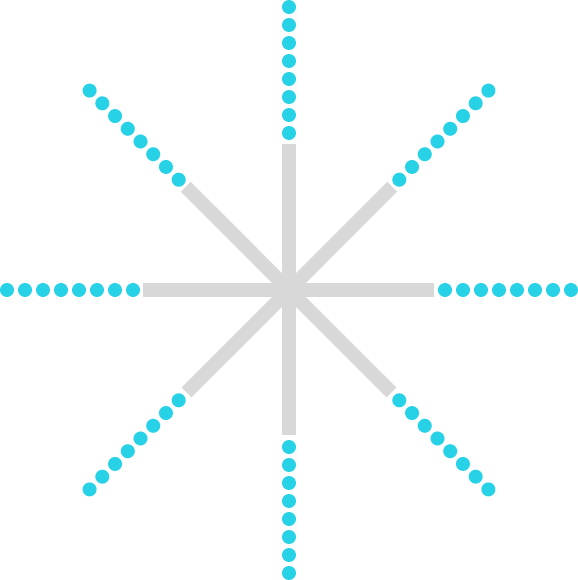Water is flowing out from the 8 holes of a star-shaped sprinkler. When the sprinkler is stationary, the water flows as indicated by the blue dots in the diagram above (when seen from above).

How does the water stream appear from the top when the sprinkler is rotated counterclockwise?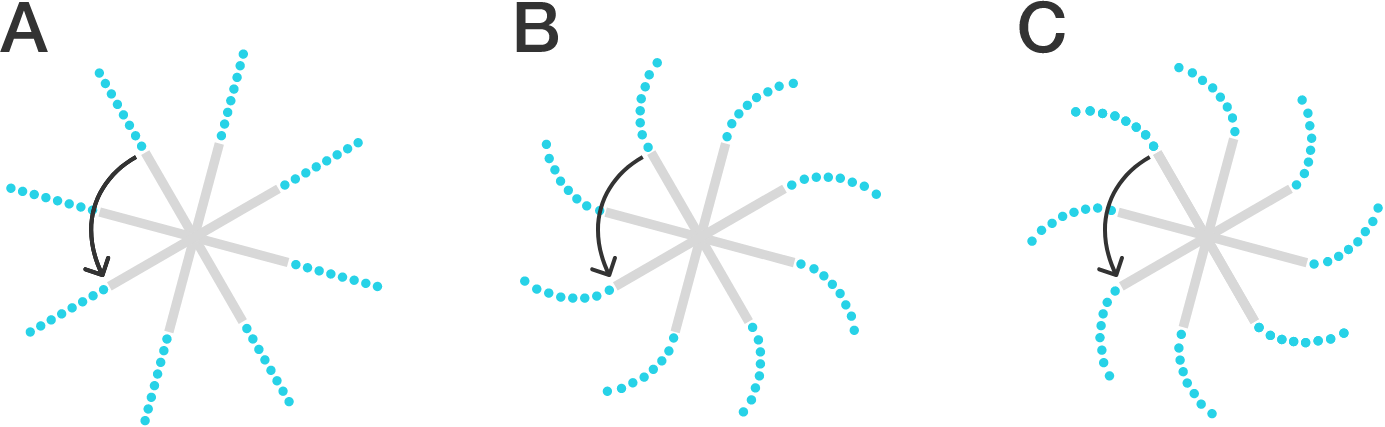Five friends (including Alice and Bob) are going to be randomly seated at a table. For which table are Alice and Bob more likely to sit next to each other?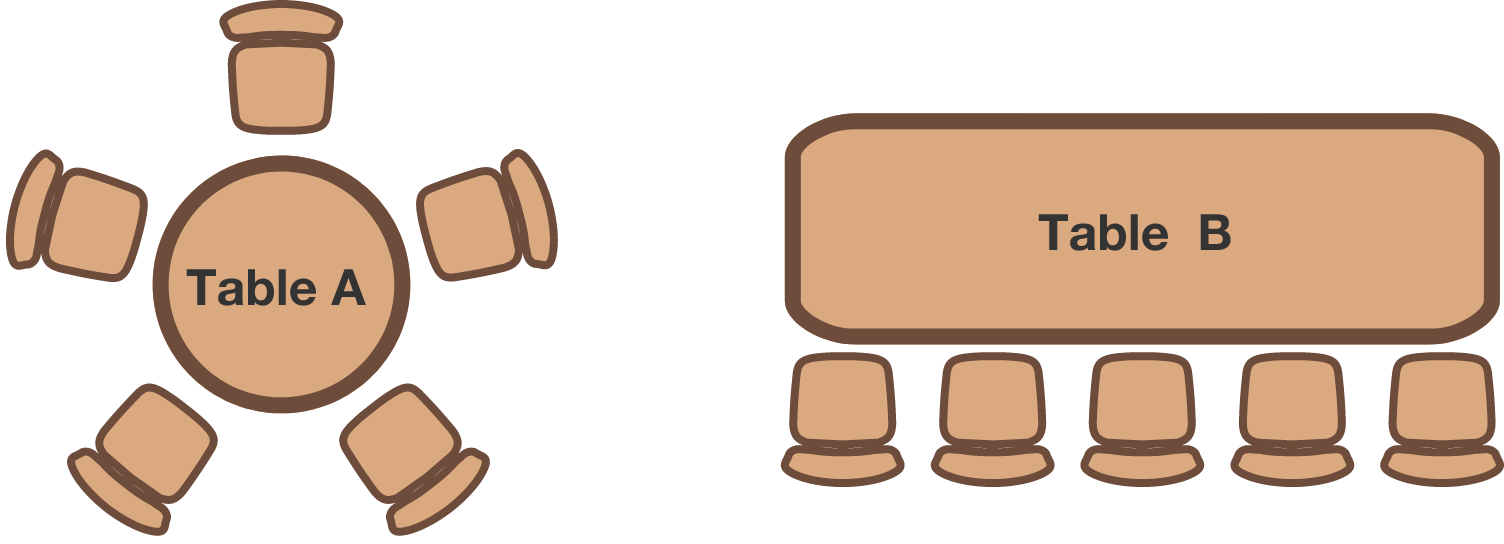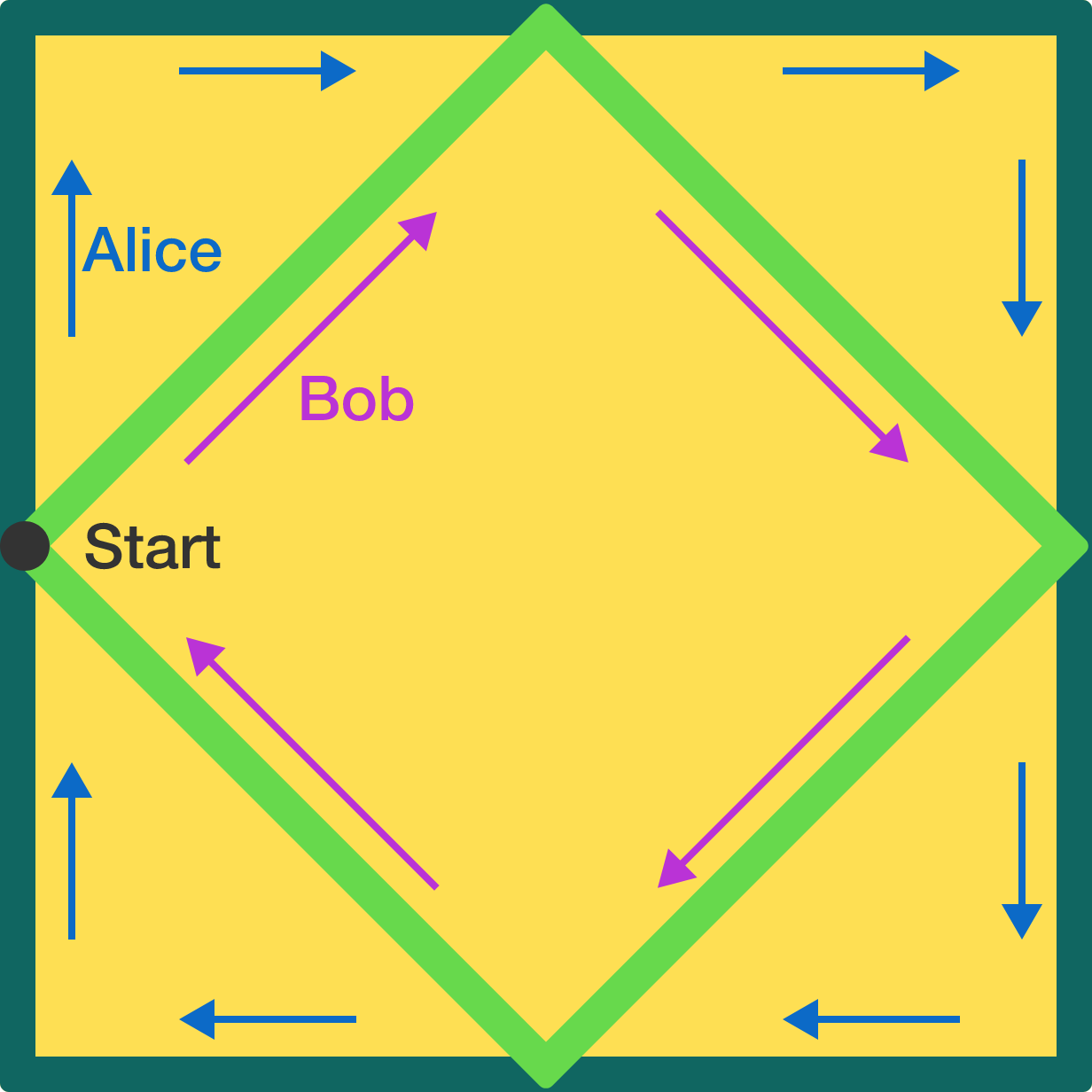Alice and Bob are standing at the point marked Start on the perimeter of a park (the large, outer square). The park also has a smaller, inner square formed by joining the midpoints of its sides.

Bob starts running around the inner square and, at the same time, Alice starts running around the outer square. Both run at the same speed.

If they keep running, will they ever be in the exact same location again at the same time?

The total area enclosed by the elephant below is 4.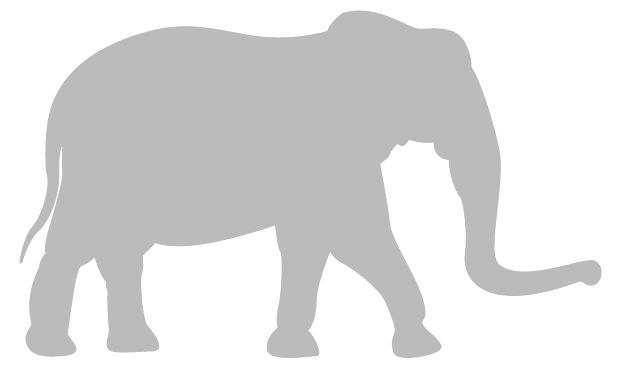Now we form a new shape by joining two of the original elephants after shrinking them widthwise by a factor of two (as shown below). Is the area enclosed by these elephants also 4?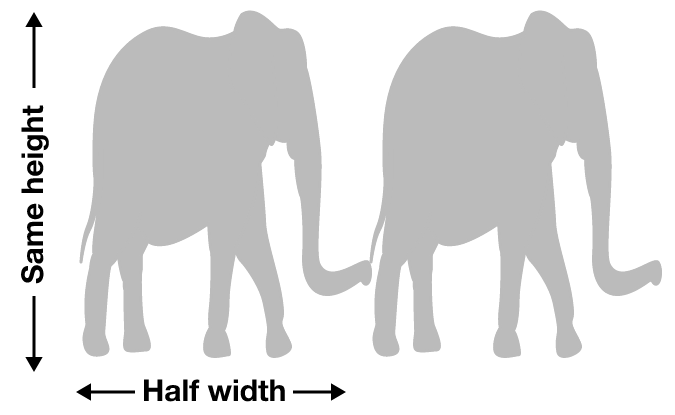$\dfrac{a+b}{c+d}=\dfrac{a-b}{c-d}$

If $a,b,c,d$ are integers, which of the following can be the value of $a\times b\times c\times d?$

×

Problem Loading...

Note Loading...

Set Loading...